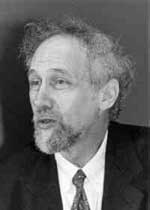OK! I asked to get side-tracked by comments so now that there is one I’d better deal with it at once. So, is there any relation between the non-commutative (algebraic) geometry based on formally smooth algebras and the non-commutative _differential_ geometry advocated by Alain Connes?
Short answers to this question might be (a) None whatsoever! (b) Morally they are the same! and (c) Their objectives are quite different!

As this only adds to the confusion, let me try to explain each point separately after issuing a _disclaimer_ that I am by no means an expert in Connes’ NOG neither in $C^*$-algebras. All I know is based on sitting in some lectures by Alain Connes, trying at several times to make sense of his terribly written book and indeed by reading the Landi notes in utter desperation.
(a) _None whatsoever!_ : Connes’ approach via spectral triples is modelled such that one gets (suitable) ordinary (that is, commutative) manifolds into this framework. The obvious algebraic counterpart for this would be a statement to the effect that the affine coordinate ring $\mathbb{C}[X]$ of a (suitable) smooth affine variety X would be formally smooth. Now you’re in for a first shock : the only affine smooth varieties for which this holds are either _points_ or _curves_! Not much of a geometry huh? In fact, that is the reason why I prefer to call formally smooth algebras just _qurves_ …
(b) _Morally they are the same_ : If you ever want to get some differential geometry done, you’d better have a connection on the tangent bundle! Now, Alain Connes extended the notion of a connection to the non-commutative world (see for example _the_ book) and if you take the algebraic equivalent of it and ask for which algebras possess such a connection, you get _precisely_ the formally smooth algebras (see section 8 of the Cuntz-Quillen paper “Algebra extensions and nonsingularity” Journal AMS Vol 8 (1991). Besides there is a class of $C^*$-algebras which are formally smooth algebras : the AF-algebras which also feature prominently in the Landi notes (although they are virtually never affine, that is, finitely generated as an algebra).
(c) _Their objectives are quite different!_ : Connes’ formalism aims to define a length function on a non-commutative manifold associated to a $C^*$-algebra. Non-commutative geometry based on formally smooth algebras has no interest in defining some sort of space associated to the algebra. The major importance of formally smooth algebras (as advocated by Maxim Kontsevich is that such an algebra A can be seen as a _machine_ producing an infinite family of ordinary commutative manifolds via its _representation varieties_ $\mathbf{rep}_n~A$ which are manifolds equipped with a $GL_n$-action. Non-commutative functions and diifferential forms defined at the level of the formally smooth algebra A do determine similar $GL_n$-invariant features on _all_ of these representation varieties at once.

### Similar Posts:

Published in featured